Custom SearchEQUIVALENT FRACTIONS It will be recalled that any number divided by itself is 1. For example, l/l, 2/2, 3/3, 4/4, and all other numbers formed in this way, have the value 1. Furthermore, any number multi- plied by 1 is equivalent to the number itself. For example, 1 times 2 is 2, 1 times 3 is 3, 1 times l/2 is l/2, etc.  These facts are used in changing the form of a fraction to an equivalent form which is more convenient for use in a particular problem. For example, if 1 in the form 2/2 is multiplied by 3/5, the product will still have a value of 3/5 but will be in a different form, as follows:Figure 4-4 shows that 3/5 of line a is equal to 6/10 of line b where line a equals line b. Line a is marked off in fifths and line b is marked off in tenths.  It can readily be seen that 6/10 and 3/5 measure distances of equal length.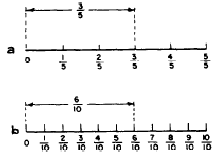Figure 4-4.-Equivalent fractions. The markings on a ruler show equivalent fractions. The major division of an inch divides it into two equal parts. One of these parts represents 1/2 The next smaller markings divide the inch into four equal parts. It will be noted that two of these parts represent the same distance as 1/2; that is, 2/4 equals 1/2.. Also, the next smaller markings break the inch into 8 equal parts. How many of these parts are equivalent to 1/2 inch? The answer is found by noting that 4/8 equals 1/2. Practice problems. Using the divisions on a ruler for reference, complete the following exercise: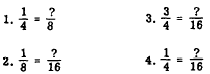Answers: 1. 2  2. 2  3. 12 4. 4 A review of the foregoing exercise will reveal that in each case the right-hand fraction could be formed by multiplying both the numerator and the denominator of the left-hand fraction by the same number. In each case the number may be determined by dividing the denominator of the right-hand fraction by the denominator of the left-hand fraction. Thus in problem 1, both terms of 1/4 were multiplied by 2. In problem 3, both terms were multiplied by 4. It is seen that multiplying both terms of a fraction by the same number does not change the value of the fraction. Since 1/2 equals 2/4, the reverse must also be true; that is 2/4 must be equal to 1/2. This can likewise be verified on a ruler. We have already seen that 4/8 is the same as 1/2 12/16 equals 3/4 and 2/8 equals 1/4. We see that dividing both terms of a fraction by the same number does not change the value of the fraction. FUNDAMENTAL RULE OF FRACTIONS The foregoing results are combined to form the fundamental rule of fractions, which is stated as follows: Multiplying or dividing both terms of a fraction by the same number does not change the value of the fraction. This is one of the most important rules used in dealing with fractions. The following examples show how the fundamental rule is used: 1. Change 1/4 to twelfths. This problem is set up as follows:The first step is to determine how many 4s are contained in 12. The answer is 3, so we know that the multiplier for both terms of the fraction is 3, as follows:2. What fraction with a numerator of 6 is equal to 3/4? SOLUTION:We note that 6 contains 3 twice; therefore we need to double the numerator of the right-hand fraction to make it equivalent to the numerator of the fraction we seek. We multiply both terms of 3/4 by 2, obtaining 8 as the denominator of the new fraction, as follows: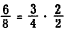3. Change 6/16 to eighths. SOLUTION: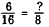We note that the denominator of the fraction which we seek is l/2 as large as the denominator of the original fraction. Therefore the new fraction may be formed by dividing both terms of the original fraction by 2, as follows:Practice problems. Supply  the missing number in each of the following:Answers: 1. 80 2. 11 3. 27 4. 36 5. 6 6. 16 REDUCTION TO LOWEST TERMS It is frequently desirable to change a fraction to an equivalent fraction with the smallest possible terms; that is, with the smallest possible numerator and denominator. This process is called REDUCTION. Thus, 6/30 reduced to lowest terms is 1/5 Reduction can be accomplished by finding the largest factor that is common to both the numerator and denominator and dividing both of these terms by it. Dividing both terms of the preceding example by 6 reduces the fraction to lowest terms. In computation, fractions should usually be reduced to lowest terms where possible. If the greatest common factor cannot readily be found, any common factor may be removed and the process repeated until the fraction is in lowest terms: Thus 18/48 could first be divided by 2 then by 3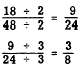Practice problems. Reduce the following fractions to lowest terms: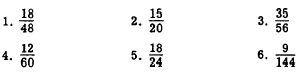Answers: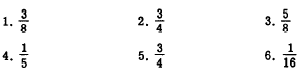Integrated Publishing, Inc. - A (SDVOSB) Service Disabled Veteran Owned Small Business